# How to deal with overplotting without being fooled

### The problem

Let me be clear, when you have gazillions of data points in a scatter plot, you want to deal with the overplotting to avoid drawing misleading conclusions.

library(dplyr)
library(Seurat)
library(patchwork)
library(ggplot2)
library(ComplexHeatmap)
library(SeuratData)
set.seed(1234)

prepare the data

data("pbmc3k")

pbmc3k
#> An object of class Seurat
#> 13714 features across 2700 samples within 1 assay
#> Active assay: RNA (13714 features, 0 variable features)
## routine processing
pbmc3k<- pbmc3k %>%
NormalizeData(normalization.method = "LogNormalize", scale.factor = 10000) %>%
FindVariableFeatures(selection.method = "vst", nfeatures = 2000) %>%
ScaleData() %>%
RunPCA(verbose = FALSE) %>%
FindNeighbors(dims = 1:10, verbose = FALSE) %>%
FindClusters(resolution = 0.5, verbose = FALSE) %>%
RunUMAP(dims = 1:10, verbose = FALSE)

Idents(pbmc3k)<- pbmc3k$seurat_annotations pbmc3k<- pbmc3k[, !is.na(pbmc3k$seurat_annotations)]

DimPlot(pbmc3k, reduction = "umap", label = TRUE)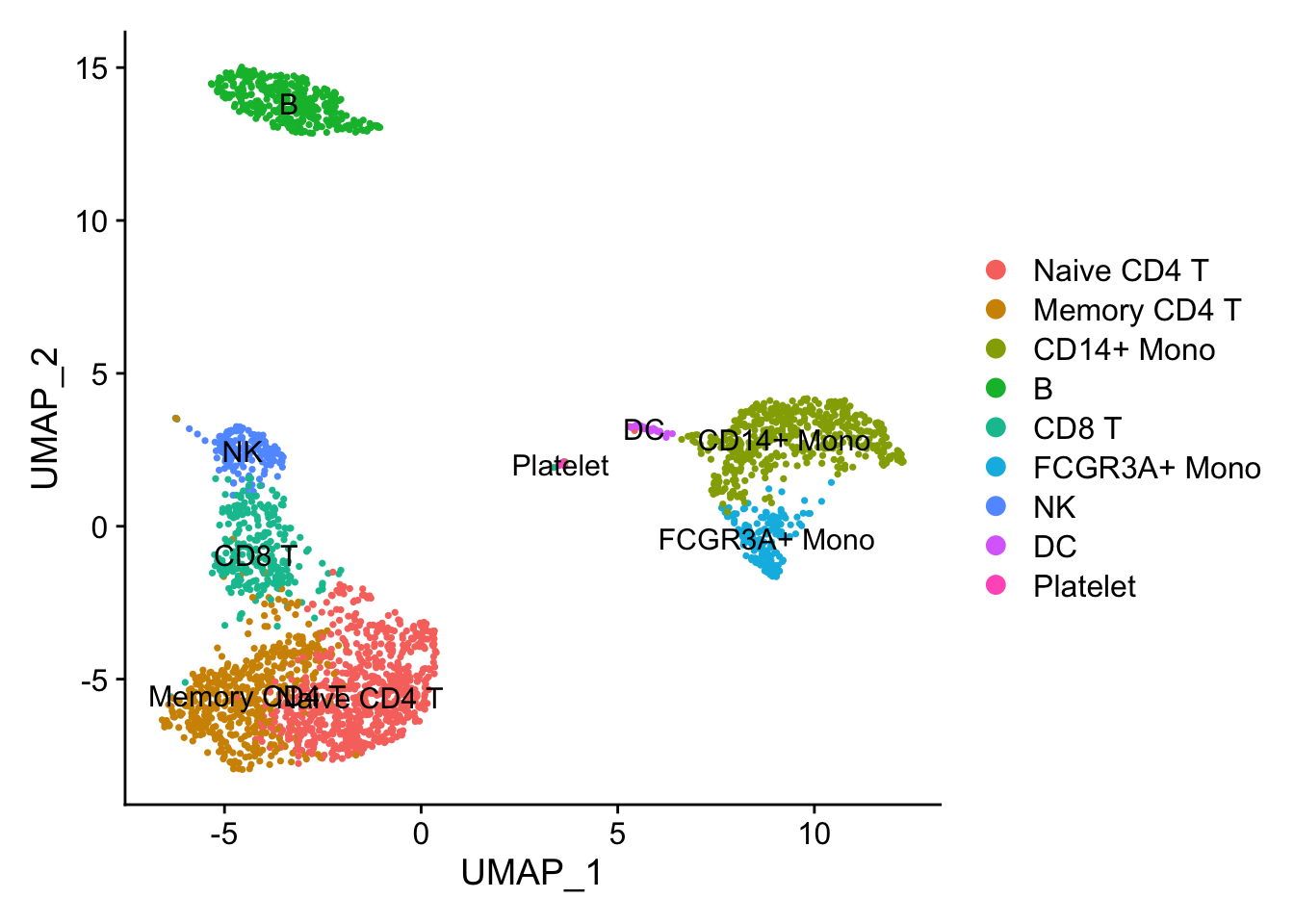### Illusion 1: dots are masked.

FeaturePlot(pbmc3k, features = c("CD4", "CD3D"))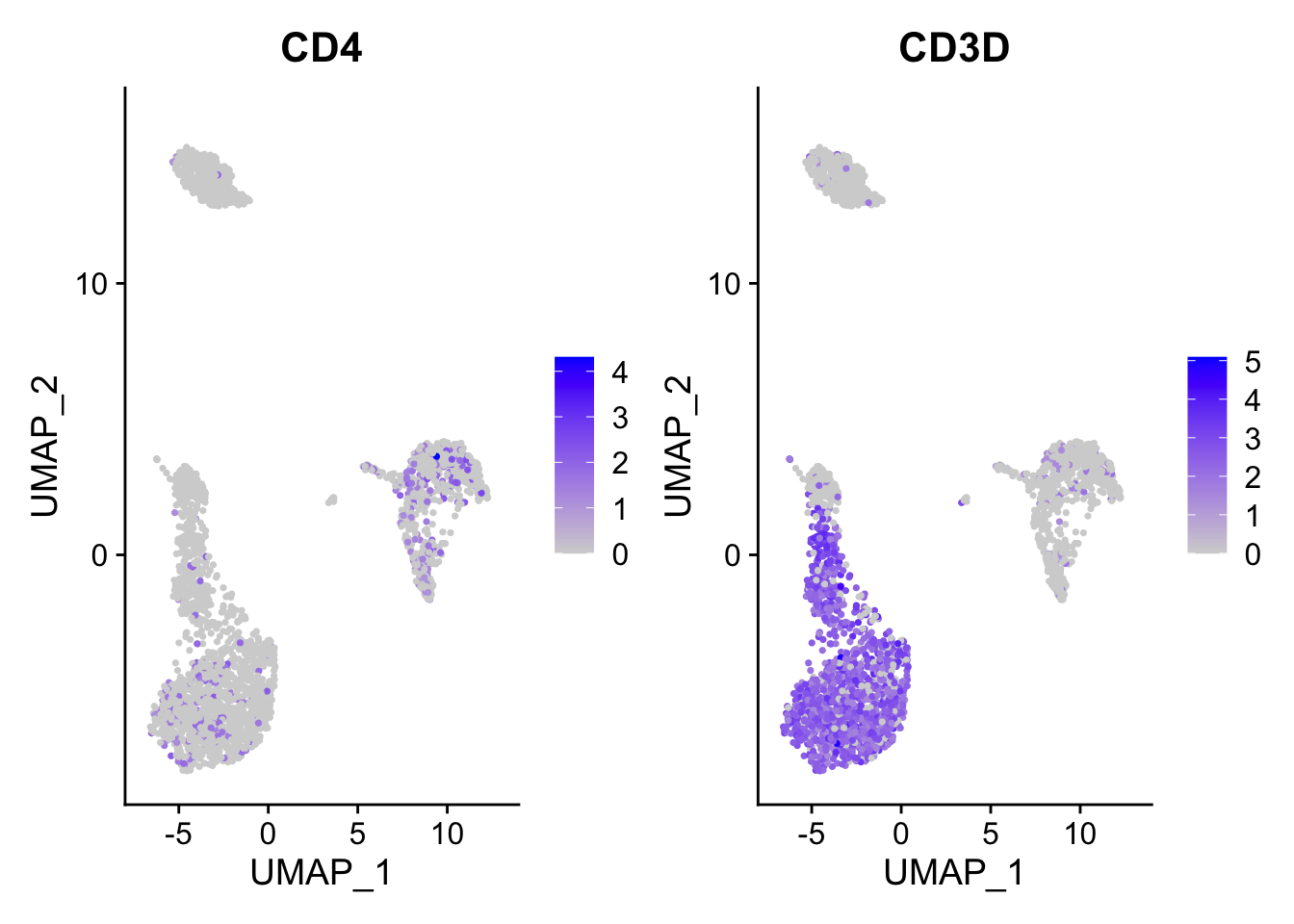ggplot2 plots the dots with the order that they show in the dataframe. When you have a lot of dots, they plot on top of each other. The blue dot can be masked by the grey dot if the grey dot/cell appears after the blue dot/cell.

FeaturePlot(pbmc3k, features = c("CD4", "CD3D"), order = TRUE)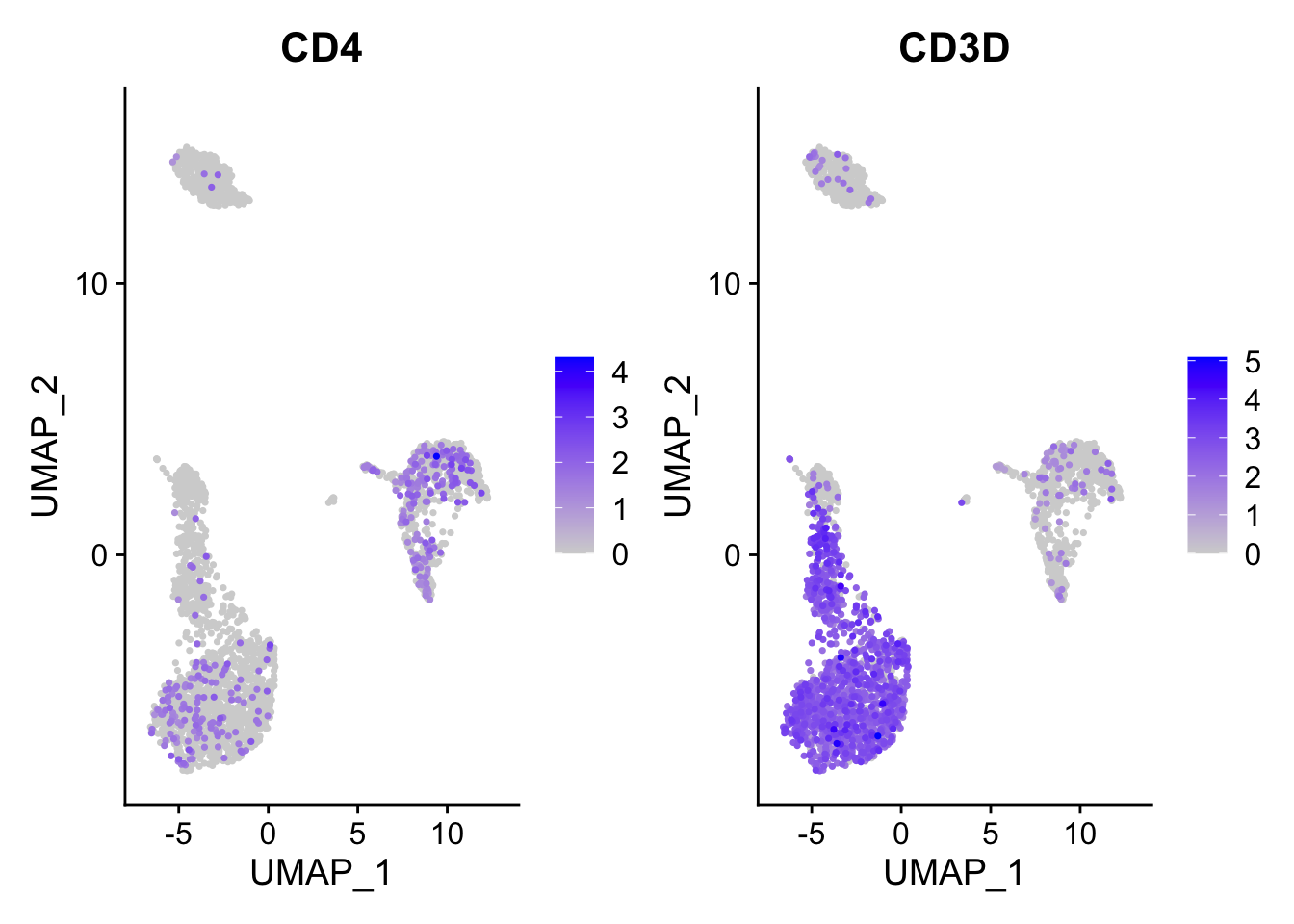You can tell that it appears both CD4 and CD3D have enhanced expression after you set the order =TRUE. Essentially, this will cause the cells with some expression of those genes plotted in the last.

Note, by default, scCustomize::FeaturePlot_scCustom set the order by TRUE. https://samuel-marsh.github.io/scCustomize/articles/Gene_Expression_Plotting.html#plot-gene-expression-in-2d-space-pcatsneumap

### Illusion2: number of dots

Only when you plot the density of the points you know where are the dots are concentrated.

p1<- FeaturePlot(pbmc3k, features = "CD3D", order = TRUE)

p2<- scCustomize::Plot_Density_Custom(seurat_object = pbmc3k, features = "CD3D",
viridis_palette= "viridis")

p1 | p2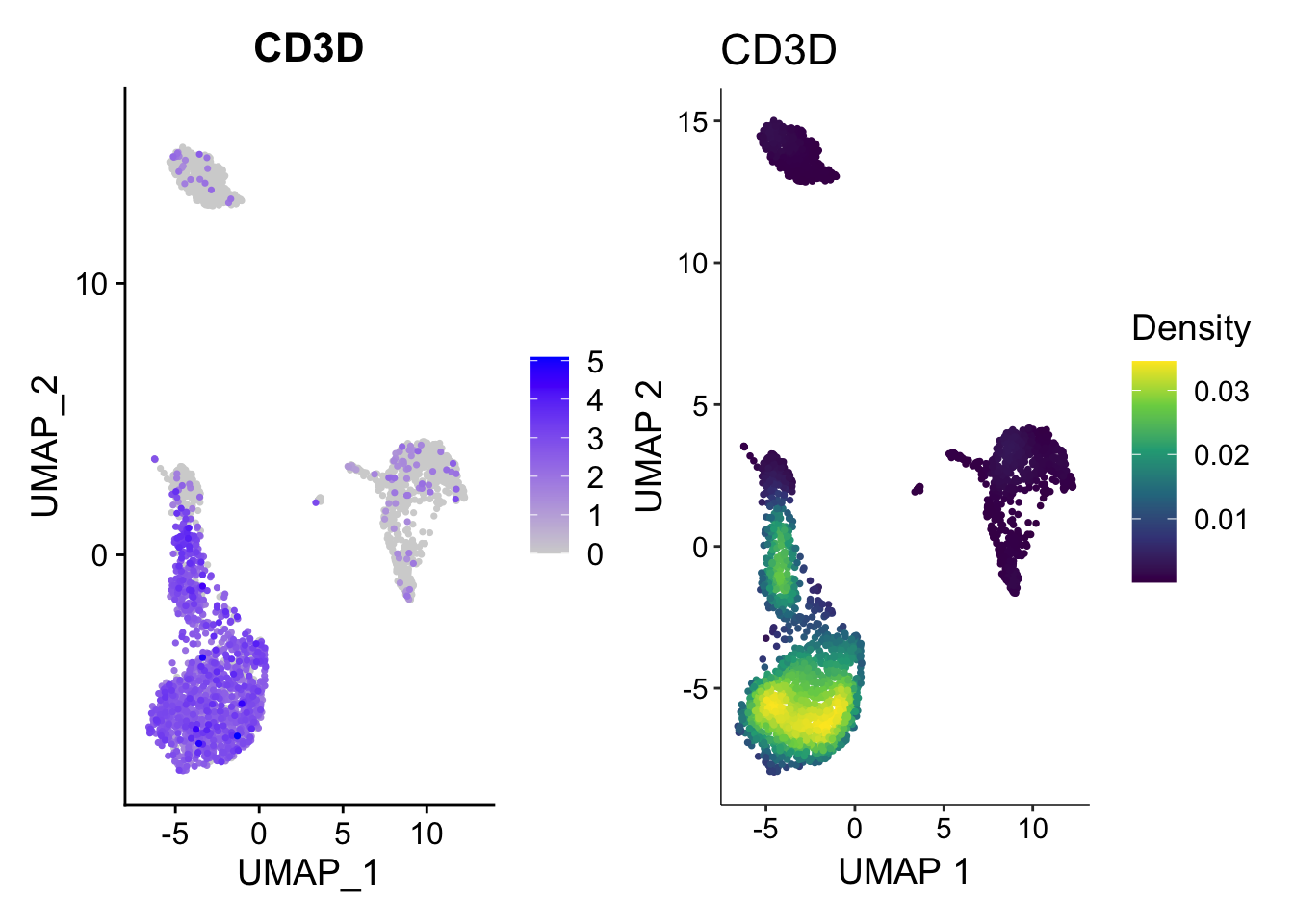How the plot on the right was made? No worries, let me show you how to plot the density plot from scratch using ggplot2.

First, some helper functions:

matrix_to_expression_df<- function(x, obj){
df<- x %>%
as.matrix() %>%
as.data.frame() %>%
tibble::rownames_to_column(var= "gene") %>%
tidyr::pivot_longer(cols = -1, names_to = "cell", values_to = "expression") %>%
tidyr::pivot_wider(names_from = "gene", values_from = expression) %>%
left_join(obj@meta.data %>%
tibble::rownames_to_column(var = "cell"))
return(df)
}

get_expression_data<- function(obj, assay = "RNA", slot = "data",
genes = NULL, cells = NULL){
if (is.null(genes) & !is.null(cells)){
df<- GetAssayData(obj, assay = assay, slot = slot)[, cells, drop = FALSE] %>%
matrix_to_expression_df(obj = obj)
} else if (!is.null(genes) & is.null(cells)){
df <- GetAssayData(obj, assay = assay, slot = slot)[genes, , drop = FALSE] %>%
matrix_to_expression_df(obj = obj)
} else if (is.null(genes & is.null(cells))){
df <- GetAssayData(obj, assay = assay, slot = slot)[, , drop = FALSE] %>%
matrix_to_expression_df(obj = obj)
} else {
df<- GetAssayData(obj, assay = assay, slot = slot)[genes, cells, drop = FALSE] %>%
matrix_to_expression_df(obj = obj)
}
return(df)
}
library(ggpointdensity)

## fetch the dataframe
df<- get_expression_data(pbmc3k, genes = "CD3D")

umap_cor<- pbmc3k@reductions\$umap@cell.embeddings  %>%
as.data.frame() %>%
tibble::rownames_to_column(var = "cell")

df<- left_join(df, umap_cor)

head(df)
#> # A tibble: 6 × 10
#>   cell            CD3D orig.ident nCount_RNA nFeature_RNA seurat_annotations
#>   <chr>          <dbl> <fct>           <dbl>        <int> <fct>
#> 1 AAACATACAACCAC  2.86 pbmc3k           2419          779 Memory CD4 T
#> 2 AAACATTGAGCTAC  0    pbmc3k           4903         1352 B
#> 3 AAACATTGATCAGC  3.49 pbmc3k           3147         1129 Memory CD4 T
#> 4 AAACCGTGCTTCCG  0    pbmc3k           2639          960 CD14+ Mono
#> 5 AAACCGTGTATGCG  0    pbmc3k            980          521 NK
#> 6 AAACGCACTGGTAC  1.73 pbmc3k           2163          781 Memory CD4 T
#> # … with 4 more variables: RNA_snn_res.0.5 <fct>, seurat_clusters <fct>,
#> #   UMAP_1 <dbl>, UMAP_2 <dbl>
p3<- ggplot(df, aes(x= UMAP_1, y= UMAP_2 )) +
geom_point(data = df %>% filter(CD3D == 0), color = "#440154FF", size = 0.6) +
ggpointdensity::geom_pointdensity(data = df %>% filter(CD3D > 0), size = 0.6) +
viridis::scale_color_viridis() +
theme_classic(base_size = 14) +
ggtitle("from scratch")

p2 | p3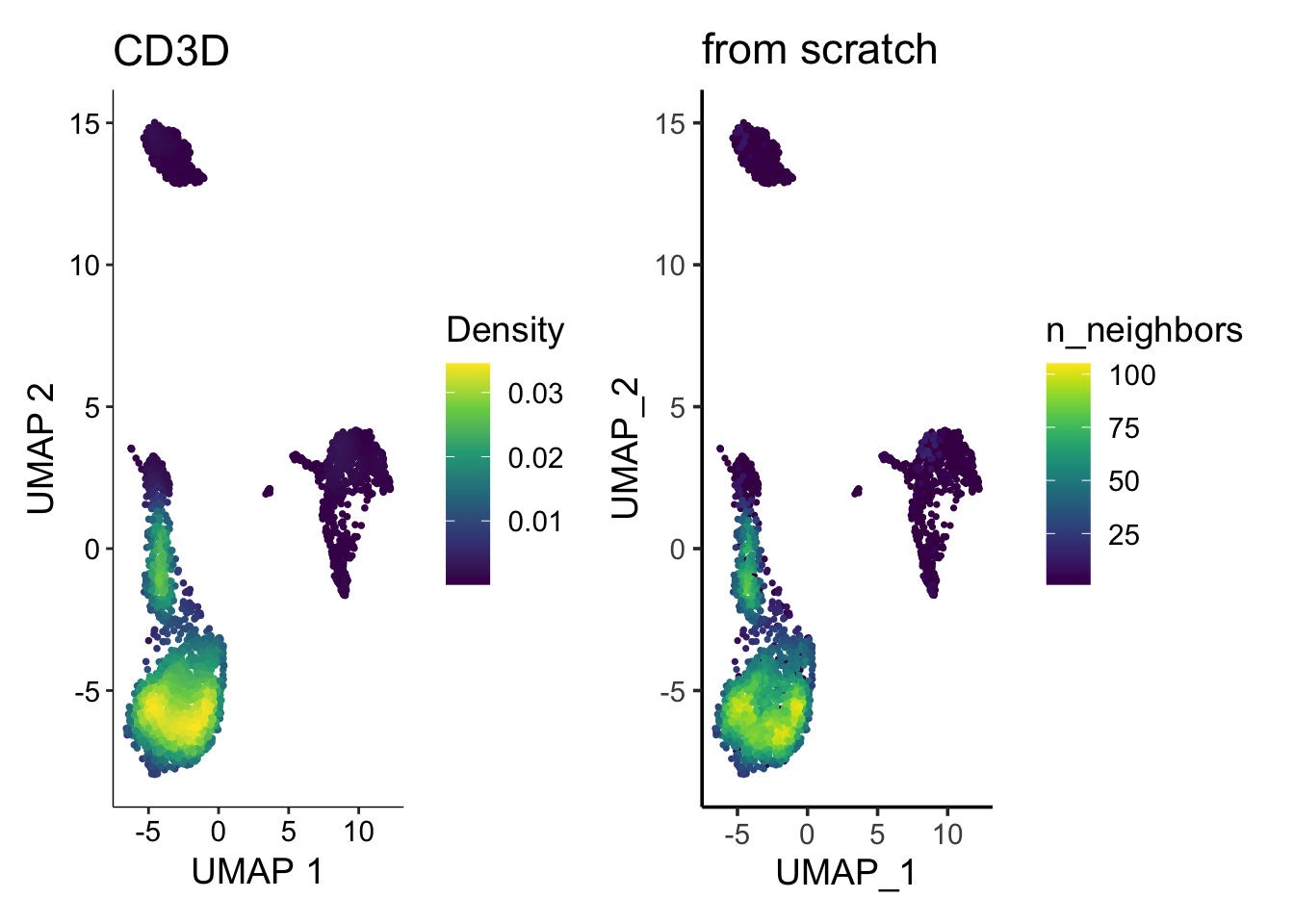They look similar enough. Note the legend is different (density vs number of neighbors), but you get the idea.

### Rastering

Have you found that when you have gazillions of points, the resulting PDF or PNG file is so big and your computer is so slow to view them?

Rastering the image come to the rescue. Let’s use https://github.com/exaexa/scattermore

library(scattermore)

ggplot(df, aes(x=UMAP_1, y= UMAP_2)) +
geom_scattermore() +
theme_classic(base_size = 14) +
ggtitle("geom_scattermore")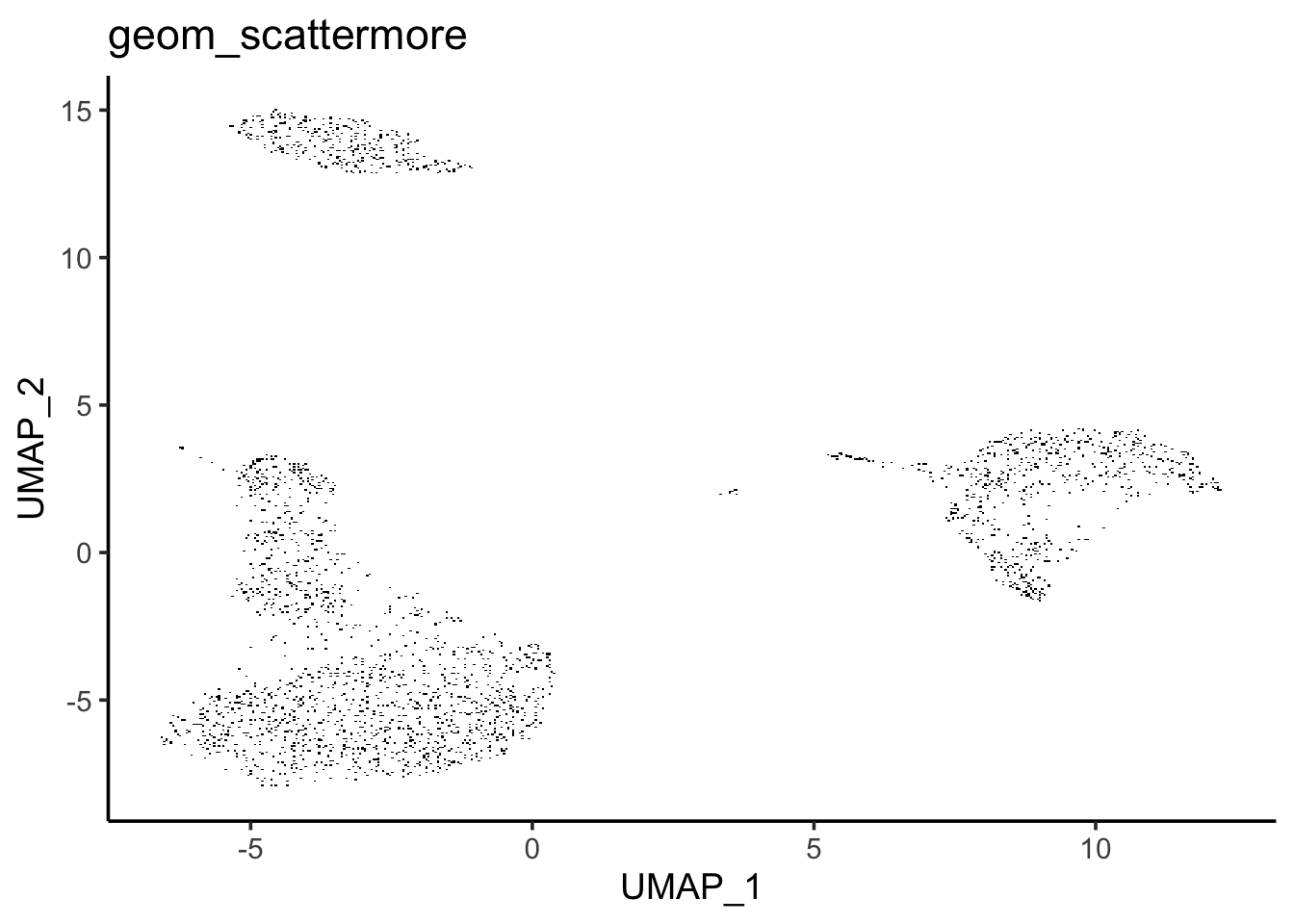You can not see the difference here. But if you zoom in the figure on your computer, you will see the rectangles of the points.

For this small dataset (only 3000 cells), you can not feel the differences of plotting speed. However, when you have millions of cells, you may want to give scattermore a try!

The same thing applies to heatmap too.